# Convert Text To Number Excel

Many times, like when we extract numbers out of string, they be text by property. You can’t do any number operations on them. Then we get the need of converting that text to number. So to convert any text to number we can take various approaches depending on the situation. Let’s have a look to those approaches…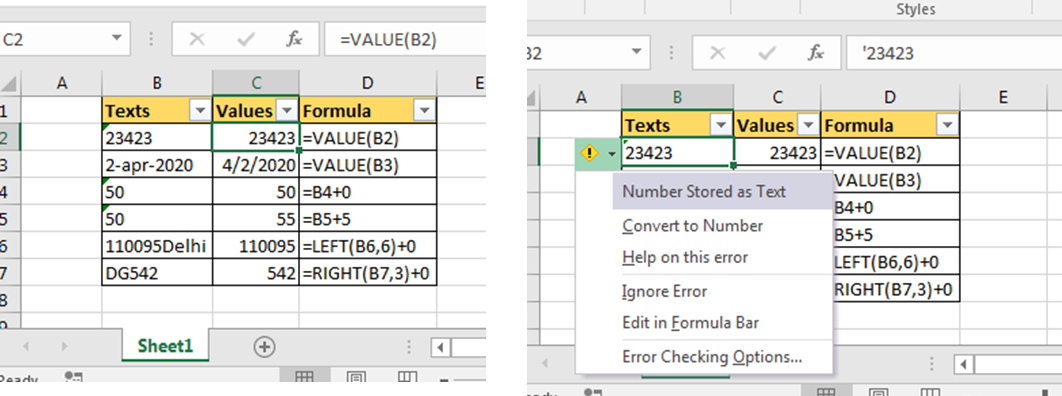Use VALUE Function to Convert Text into Number
So if you downloaded sum data from a website, its common to get some numbers as text format special dates. Just wrap these text into VALUE function. It will return a pure number.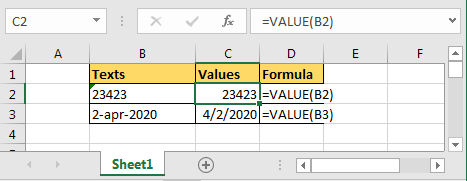VALUE function Syntax

=VALUE(text)

So to convert a text in cell B2 into number write this VALUE formula.

=VALUE(B2)

Add 0 to text to Convert Text into Number
If you add any number using + operator to text formated number, it will convert the text to number and then add the given number to converted number. Finally result will be a number.

For example if there is text formated number in B4, then just add 0 to convert string to number.

=B4+0

Result will be a number for sure.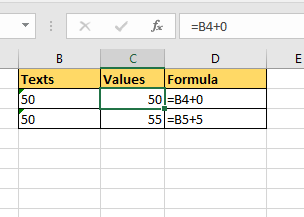As I told you in the beginning that this problem occurs when we extract number from string. So if you want to the extracted text to be number just add 0 in the end.

For example i am extracting citycode from text B6. I extracted it using LEFT function. But its not a number so I added a 0 in the formula itself.

=LEFT(B6,6)+0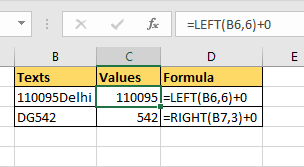Using Excel Notification to Convert Text into Number
Whenever a number is in form of a text, excel notifies you by showing a green corner of cell.
When you click on cell it shows a small exclamation icon at the left corner of cell.
When you click on it, it shows an option of >Covert to Number. Click on it and text will be converted to number.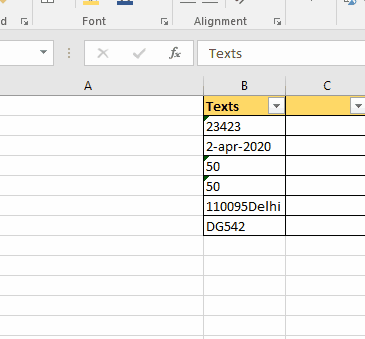So yeah, these are the ways to convert string to text in Excel. Now you know how easy converting text to numbers in excel is.

Terms and Conditions of use

The applications/code on this site are distributed as is and without warranties or liability. In no event shall the owner of the copyrights, or the authors of the applications/code be liable for any loss of profit, any problems or any damage resulting from the use or evaluation of the applications/code.